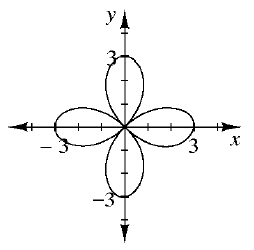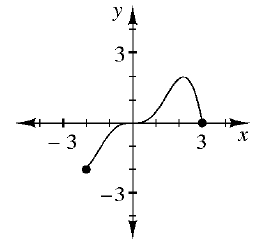### Home > INT1 > Chapter 6 > Lesson 6.3.3 > Problem6-108

6-108.

Examine the graphs below. Decide if each graph represents a function. Then describe the domain and range of each one.

1.Is there more than one output value for a single input value?
If so, it is not a function.

Determine the possible x-values to find the domain and the possible $y$-values to find the range.

It is not a function.
$R: − 3 ≤ x ≤ 3$

1.Is there more than one output value for a single input value?
If so, it is not a function.

Determine the possible $x$-values to find the domain and the possible $y$-values to find the range.

It is a function.
$D: −2 ≤ x ≤ 3$